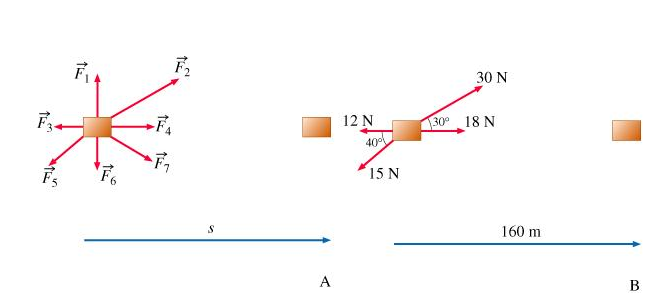# Problem: Learning Goal: To be able to calculate work done by a constant force directed at different angles relative to displacementIf an object undergoes displacement while being acted upon by a force (or several forces), it is said that work is being done on the object. If the object is moving in a straight line and the displacement and the force are known, the work done by the force can be calculated asW= F*s=/F/ /s/ cosθwhere W  is the work done by force F on the object that undergoes displacement s directed at angle θ relative to F.Note that depending on the value of cosθ, the work done can be positive, negative, or zero.In this problem, you will practice calculating work done on an object moving in a straight line. The first series of questions is related to the accompanying figure. (Intro 1 figure)Find the work W done by the 30-newton force.Use two significant figures in your answer. Express your answer in joules.

🤓 Based on our data, we think this question is relevant for Professor Narayanan's class at FIU.

###### FREE Expert Solution

Work done:

The 30 N force makes an angle θ = 30° relative to the direction of motion.###### Problem Details

Learning Goal: To be able to calculate work done by a constant force directed at different angles relative to displacement

If an object undergoes displacement while being acted upon by a force (or several forces), it is said that work is being done on the object. If the object is moving in a straight line and the displacement and the force are known, the work done by the force can be calculated as
W= F*s=/F/ /s/ cosθ

where W  is the work done by force F on the object that undergoes displacement s directed at angle θ relative to F.

Note that depending on the value of cosθ, the work done can be positive, negative, or zero.

In this problem, you will practice calculating work done on an object moving in a straight line. The first series of questions is related to the accompanying figure. (Intro 1 figure)Find the work W done by the 30-newton force.